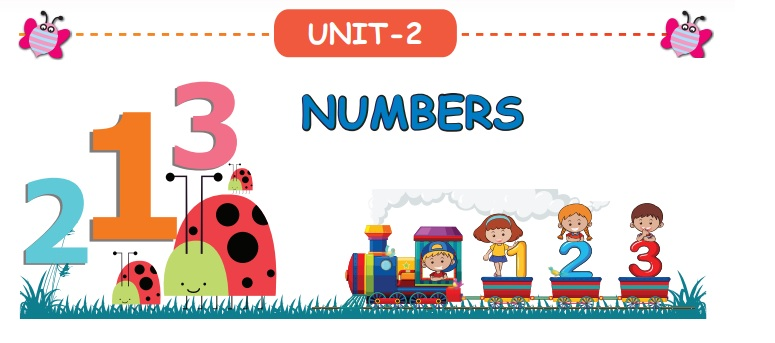Home | | Maths 3rd Std | Numbers

# Numbers

1. Look at the picture and answer the following. 2. Fill in the blanks in each of the figures with missing numbers. 3. Complete the given facts by placing + for addition and − for subtraction.

UNIT− 2

NUMBERSTravel Through1. Look at the picture and answer the following.2. Fill in the blanks in each of the figures with missing numbers.

a) 51 52 53 54 55 56 57 58 59 60

b) 74 75 76 77 78 793. Complete the given facts by placing + for addition and − for subtraction.

9 __ 3 = 12 Answer: 9 + 3 = 12

80 __ 11= 91 Answer: 80 +11= 91

56 __ 21= 35 Answer: 56 21= 35

92 __ 20 = 72 Answer: 92 20 = 72

12 __ 3 = 9 Answer: 12 3 = 9

75 __ 17 = 92 Answer: 75 + 17 = 92

Tags : Term 1 Chapter 2 | 3rd Maths , 3rd Maths : Term 1 Unit 2 : Numbers
Study Material, Lecturing Notes, Assignment, Reference, Wiki description explanation, brief detail
3rd Maths : Term 1 Unit 2 : Numbers : Numbers | Term 1 Chapter 2 | 3rd Maths